# Diversity-Weighted Equity Indexes

April 01, 1999

Diversity weighting, the author's alternative to capitalization weighting, should add 45 basis points per year to returns on average, by his calculation. After 1998, when the U.S. equity markets became concentrated in a small number of the largest companies to a historic degree, this method might have even greater potential value if there is regression toward the historical mean.Capitalization weighting is not the only structure for equity indexes. Diversity weighting is a new type of passive indexing that will increase long-term investment returns under reasonable assumptions on long-term market stability. Diversity weighting is based on market diversity, a mathematical measure of the level of concentration of capital in the market. For a diversity-weighted index there is less concentration of capital in the largest companies than for the corresponding capitalization-weighted index holding the same stocks. Given the current market conditions, this might be a good time to include diversity weighting as part of a passive mix.

Diversity-weighted indexing is a dynamic strategy in the sense that the stock holdings are adjusted from time to time as stock prices change. The strategy depends on stochastic calculus, a powerful mathematical methodology used for analyzing systems of time-dependent random processes. Stochastic calculus has now been established as the basis of most of modern mathematical finance (see Karatzas and Shreve, 1998). Capitalization-weighted indexing is a static strategy in the sense that the stock holdings are not adjusted as stock prices change. Practically all institutional equity index funds follow capitalization-weighted indexes that were developed before stochastic calculus had been applied to finance.

Diversity weighting uses no form of stock selection, optimization, or derivatives, so diversity-weighted indexing does not fall into the category of enhanced indexing. Diversity weighting gives investors a practical alternative to capitalization weighting for passive investment.

DIVERSITY

Diversity is a measure of the spread of capital across an equity market (see Fernholz, 1999a). Diversity is higher when capital is spread more evenly among the stocks in the index, and is lower when capital is more concentrated among a few large stocks. Changes in diversity measure the ebb and flow of capital between the larger and smaller stocks. Diversity decreases when capital flows into the larger stocks, resulting in more concentration of capital in fewer stocks. Diversity increases when capital ebbs back into the smaller stocks, resulting in a less concentrated distribution of capital. Consider the capital distribution curve in Figure 1.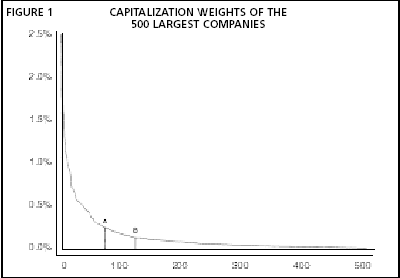It shows the capitalization weights of the largest 500 stocks in the U.S. equity market at the beginning of 1998. The weights are in decreasing order from the largest at about 2.5% on the left, to number 500 at about .03% on the right. Diversity provides a mathematical measure of the relative level of concentration of capital distributed along the curve, and diversity varies over time according to the movement of capital between large and small stocks.

Figure 2 shows the variation in market diversity over the period from 1927 to 1998, calculated using monthly data from the Center for Research in Securities Prices (CRSP) at the University of Chicago. As can be seen from the chart, diversity is variable over the short term, but has been mean-reverting over the long term. The low points on the diversity curve, indicating extreme concentration of capital in the large stocks, occurred during the Great Depression in 1932, the 'Nifty Fifty'' era in 1974, and now. It would seem unlikely that diversity would indefinitely continue to trend downwards, for this would result in the concentration of almost all the capital into a few companies. Hence, diversity seems likely to continue its cycle, increasing over some periods and decreasing over others.

The mean-reverting nature of the variation in diversity implies that the capital distribution curve in Figure 1 is fairly stable over the long term. Let us suppose for a moment that a period of time passes and the stock prices change, but this capital distribution curve remains completely unchanged. This means that the stock price movement can only rearrange the order of the stocks: i.e., the names are reassigned to different weights. Suppose that the two stocks A and B in the chart switch places, and nothing else moves. For a capitalization-weighted index, this has no effect, since the two stocks merely replace each other. Now it is clear that if the stocks switch places, then B must have a higher return than A. Hence, if we had been able to shift some capital from A to B, we would have outperformed a capitalization-weighted index. But how can we predict which stocks are going to switch places? It turns out that this question is irrelevant, because with diversity weighting we can exploit the continual shifting of positions along the capital distribution curve without forecasting the relative movement of the stocks.

Of course, the assumption that the capital distribution curve could remain totally fixed is unrealistic, but as we shall see in the next section, the theory of diversity weighting takes into account the changes in the distribution curve, and separates the effect of these changes from the effect of stock movements along the curve. Thus, the example we have considered captures the essential mechanism that drives diversity weighting,DIVERSITY-WEIGHTED INDEXING

It was proven in Fernholz (1999b) that any mathematical measure of index diversity can be used to construct a set of dynamic portfolio weights. These weights are called diversity weights and the portfolio they represent is called a diversity-weighted index. A diversity-weighted index holds exactly the same stocks as the capitalization-weighted index it is derived from, but the stocks are weighted differently. Relative to the capitalization-weighted index, the diversity-weighted index is under weighted in the larger stocks and over weighted in the smaller stocks.

Central to the relationship between diversity weighting and capitalization weighting is an equation which states that Relative return = Variation in diversity + Kinetic differential + Differential dividend rate.

This relationship holds for any diversity-weighted index and the capitalization-weighted index composed of the same stocks. The equation is essentially an accounting identity that decomposes the relative return into three separate components. The variation in diversity is the change in the value of the measure of diversity and the kinetic differential is the added return generated by the continuous random movement of the stock prices, as when stocks Aand B switched places in the example of the previous section. The differential dividend rate accounts for the fact that the two versions of the index are likely to have different dividend rates. It should be noted that all the quantities in equation (1) are treated logarithmically.

In order to apply the theory to actual markets, certain adjustments are necessary. The variation in diversity in equation (1) must be adjusted so that it includes only variation caused by capital gains or losses in the stocks. Not included is variation in diversity that results from changes in the composition of the S&P 500, or from corporate actions such as mergers, acquisitions, or spin offs. These adjustments have the same effect as changing the 'divisor'' of an index. Also, an adjustment to the kinetic differential must be made for leakage, the systematic attrition of small stocks that are dropped from the index and replaced by others.

We shall consider a simulation of the performance of a diversity-weighted market index over the 72-year period from 1927 to 1998 using monthly CRSP data. The market included all the U.S. exchange traded and NASDAQ stocks, after the removal of REITs, ADRs, closed-end funds, and stocks that never exceeded .005% of market weight: there were about 7000 stocks in this market. Figures 2 through 5 show the cumulative values of the four quantities in equation (1) over the 72-year period. Note that these results represent an index and not an actual portfolio, so there are no transaction costs. Figures 2, 3, and 4 can be seen to 'add up'' to Figure 5, as equation (1) implies.

The charts show that almost all the short-term volatility in the relative return between the two indexes was caused by the variation in diversity, while almost all of the superior long-term performance of the diversity-weighted index was due to the kinetic differential. Over the whole period, the logarithmic relative return of the diversity-weighted index averaged about 1.05% more than that of the capitalization-weighted index. Let us note that this annual mean is measured logarithmically, and the corresponding arithmetic value would be increased by the average appreciation of the index over the period. For example, average market appreciation of 10% a year would have produced a mean annual arithmetic relative return of about 1.16%. The diversity-weighted version of the index had a Sharpe ratio (see Sharpe, 1975) of .465 over the period considered, compared to .449 for the capitalization-weighted version.

The variation in diversity in Figure 2 represents the cumulative logarithmic variation in the diversity of the market. It has behaved as a mean-reverting process with an annual standard deviation of about 4.8%. Mean-reverting processes are noisy in the short term, but exhibit long-term stability. The annual mean of the variation in diversity was about -.41%, statistically insignificant compared to the standard deviation. It should also be noted that diversity was high in 1927, but it hit a record low at the end of 1998.

The fact that the variation in diversity has been mean-reverting in the past does not necessarily mean that it will be in the future. Nevertheless, a long-term downward drift in diversity is unlikely because the concentration of almost all capital into a few giant companies would probably not be an efficient configuration for the national economy. Moreover, there are antitrust laws. The mean-reverting nature of the variation in diversity causes the two versions of the index to have essentially the same long-term volatility.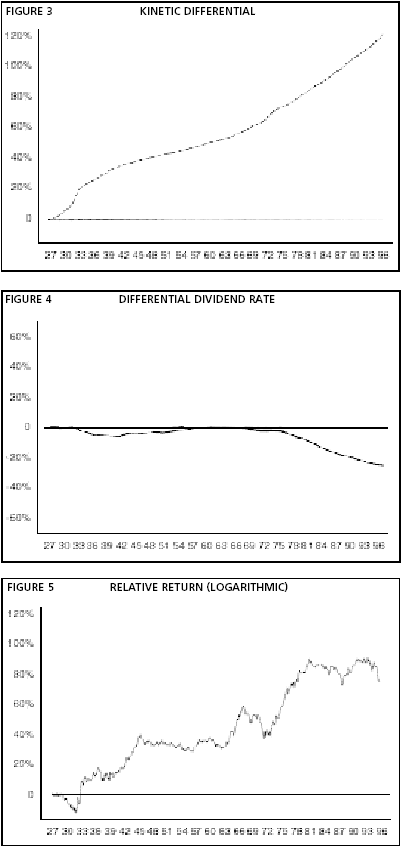The kinetic differential, shown in Figure 3, is the essence of diversity weighting. The kinetic differential is close to a pure trend process, as indicated by the smoothness of the curve. Over the period studied, the kinetic differential averaged about 1.66% a year, logarithmic. With 10% annual market appreciation, this would contribute about 1.83% a year to the relative arithmetic return. The kinetic differential is generated by the continuous random motion of the stocks in the market, and is not dependent on any shift in capital from large to small stocks. The ebb and flow of capital among stocks manifests itself in the variation in diversity, not in the kinetic differential.

Figure 4 shows the differential dividend rate between the two indexes. It is clear from Figure 4 that dividend payments by the larger stocks were not nearly great enough to offset the kinetic differential. The average annual contribution of the differential dividend rate over the period was about -.19%,but after 1980 the difference increased to about -.56%. However, even after 1980 the differential dividend rate would have had to have been about four times greater than kinetic differential.

If corporations repurchase their own shares, this can have an effect similar to the payment of dividends. Cole (1996) studied this practice over the period from 1975 to 1995, and found that this could increase the effective dividend rate of the S&P 500 Index by as much as 80 basis points a year in some of the later years. It is not clear how much share repurchases might have changed the differential dividend rates in our simulation. In another study, Shulman (1997) showed that if the issuance of options and new shares is also considered, the effective dividend rates are actually reduced. Again, the effect on our differential dividend rates is not clear. In any case, the change in diversity in Figure 2 is adjusted for corporate actions that change the number of shares of stock outstanding, and with this adjustment, the repurchase or issuance of shares need not be considered in the differential dividend rate.

A DIVERSITY-WEIGHTED S&P 500 INDEX

As a practical application of diversity weighting, INTECH, an institutional equity manager, created the INTECH Diversity Index as a benchmark for the diversity-weighted version of the S&P 500 Index (see Fernholz, Garvy, and Hannon (1998) for details). The weights for the diversity-weighted and the capitalization-weighted versions of the S&P 500 can be seen in Figure 6. The weights are from the month of May 1999. In the chart, the weights of the stocks are given in percent, with the stocks sorted by decreasing capitalization, the same as in Figure 1.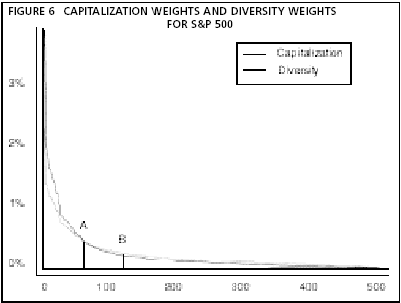Figure 6 shows that the diversity weights are lower than the capitalization weights for the largest stocks, and higher for the smallest. For the largest stock, Microsoft, the capitalization weight is about 3.6% while the diversity weight is about 2.2%. To move from capitalization weighting to diversity weighting would require about 13% turnover of the portfolio.

Fernholz, Garvy, and Hannon (1998) estimated the annual portfolio turnover for the diversity-weighted S&P500 to be about 11%. In comparison, the capitalization-weighted S&P500 Index has about 6% annual turnover, and the Russell 1000 Index about 8%. For all three indexes, most of the turnover is generated by stocks entering or leaving the index; diversity weighting requires periodic shifts in shareholdings, which accounts for its greater turnover. None of these turnover numbers represents a practical impediment to fund management. INTECH has been managing an actual portfolio replicating the diversity-weighted S&P 500 since July of 1996. Over the three-year period average annual return (before fees) was 27.43% or (after fees up to 0.09%) 27.34% compared to 27.40% for the INTECH Diversity Index, the benchmark. The portfolio was small, in the range of about \$50 million to \$200 million, and it is likely tracking error would have been less for a larger portfolio.

The diversity-weighted S&P 500 was constructed to generate an average of about .45% kinetic differential annually. Fernholz, Garvy, and Hannon (1998) estimated dividend rates for the two versions of the index are currently about the same, so this would imply about .45% of annual logarithmic outperformance for diversity weighting. [When S&P 500 diversity increases or decreases equation (1) implies this will directly affect relative performance.] This effect is summarized in Table 1.

 TABLE 1 EXPECTED PERFORMANCE OF A DIVERSITY-WEIGHTED INDEX ASSUMING .45% ANNUAL KINETIC DIFFERENTIAL Market Environment Contribution of Kinetic Differential Expected Performance versus Cap-Weighted Index Increasing diversity +.45% Greater than .45% over performance Level diversity +.45% .45% over performance Decreasing diversity +.45% Likely under performance

The annual standard deviation of the relative return between the diversity-weighted and the capitalization-weighted versions of the S&P 500 Index is about 125 basis points, but the total volatility of the two versions of the index is essentially the same (see Fernholz, Garvy, and Hannon 1998). Over some periods the return on one version of the index has a slightly greater standard deviation; over other periods the return on the other one does. For the sake of comparison, the annual standard deviation of the relative return of the Russell 1000 Index versus the S&P 500 Index is about 155 basis points.

Diversity weighting also offers an opportunity for asset allocation between large-stock indexes. In times of low diversity, the capitalization-weighted S&P 500 can become quite concentrated into the largest stocks. Diversity weighting has lower weights for the largest stocks, so it offers investors some protection against this concentration of assets. Moreover, if the variation in diversity of the index continues to be mean-reverting, allocating more funds to diversity weighting when diversity is low could significantly improve returns. It could be conjectured that investment in a broader market index such as the Wilshire 5000 might be advisable during periods of low diversity. However a comparison of the performance of the diversity-weighted S&P 500 and the capitalization-weighted Wilshire 5000 appears to favor the diversity-weighted index, as summarized in Table 2. Moreover, we know from the accounting identity expressed by equation (1) that a diversity-weighted index will capture increases in diversity, whereas broad market index might capture them.

 TABLE 2 COMPARATIVE PERFORMANCE STATISTICS FROM 1971 TO 1998 Average Annual Return Annual Std. Dev. Sharpe Ratio Diversity-Weighted S&P 500 15.43% 16.44% .51 Wilshire 5000 14.99% 16.89% .47

At the moment, the only diversity-weighted index available is the diversity-weighted S&P500. However, diversity weighting can be used on any large-stock index. Hence, investors could benefit from diversity weighting in international as well as domestic U.S. markets. (Diversity weighting of small-stock indexes does not appear to be practical, due to leakage of large stocks.)

CONCLUSION

Diversity-weighted indexing offers investors a new alternative: a passive large-stock strategy with an expected return close to that of enhanced indexing. The fact that diversity weighting is supported by a rigorous mathematical theory implies that this enhanced performance is not merely a random anomaly. We have seen that an adversity-weighted index will have a significant performance advantage over a capitalization-weighted index under conditions of level or increasing diversity unless large companies pay enough dividends to offset the kinetic differential. In fact, the dividends of large companies have not been much higher than those of smaller companies, and not nearly high enough to eliminate this differential. If rational investors can be expected to eventually take advantage of opportunities to improve performance, then diversity weighting should become an important passive strategy.

The greatest advantage of capitalization weighting is that it enjoys the status of 'safe harbor'' for passive equity investment. The greatest disadvantage of diversity weighting is that it does not have this status. If that situation had been the reverse, and diversity-weighted indexing were the safe harbor, what possible rationale could there be to move to capitalization weighting under current market conditions?

APPENDIX: MATHEMATICAL RESULTS

The mathematical theory developed in Fernholz (1999b) shows that a wide class of measures of diversity give rise to portfolios with characteristics similar to those of the diversity-weighted market index presented in Figures 2 through 5. For any such portfolio, there is an equation of the form Relative return = Variation in diversity + Kinetic differential + Differential dividend rate. in which the terms depend on the measure of diversity used. For the portfolios that interest us, the measure of diversity is the function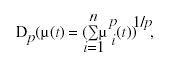where n is the total number of stocks in the index, µi(t), for which i = 1,..., n, are the capitalization weights of each of the stocks at time t, and p is a constant between 0 and 1. For the diversity-weighted S&P 500, the value of p used is .76, while the value p = . 5 was used for the market index we simulated. The diversity weights pi(t) generated by Dp are given by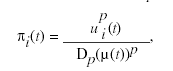for i = 1,..., n. Thus, the diversity weights are proportional to the p-th power of the capitalization weights, and this tends to under weight the larger stocks and over weight the smaller stocks.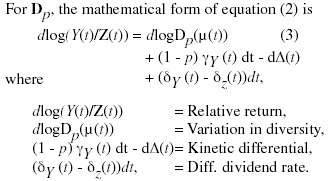Equation (3) is technically an Itô stochastic differential equation, where, intuitively, the symbol 'd,'' which occurs in each of the three expressions above, measures the change in a value over a short period of time, dt. In this equation, Y(t) represents the value of the diversity-weighted index and Z(t) represents the value of the capitalization-weighted index at time t, in each case with dividends reinvested. The equation is valid for all diversity-weighted portfolios that use the Dp diversity measure.

The components in the kinetic differential are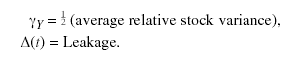The differential dividend rate is simply the dividend rate of the diversity-weighted index minus the dividend rate for the capitalization-weighted index. The average relative stock variance is the weighted average, using the diversity weights, of the relative variances of individual stock returns versus the diversity-weighted index. The term gamma g Y is a measurement of the random movement of the stock prices that generates the kinetic differential. For the diversity-weighted market index, g Y averaged about 4.10% a year from 1927 to 1998, so when multiplied by (1-p), this component contributed an average of about 2.05% a year to the relative return. The leakage measures the loss in relative return due to stocks that decline and are subsequently dropped from the index and replaced by others. For the diversity-weighted market index from 1927 to 1998 this loss averaged about .39% a year.

Mathematical proofs for this material can be found in Fernholz (1998, 1999b).

REFERENCES

Avellaneda, M. (Ed.) (1999). Quantitative Analysis in Financial Markets. River Edge, NJ: World Scientific.

Cole, K., J. Helwege, and D. Laster (1996, May/June). Stock market valuation indicators: Is this time different? Financial Analysts Journal, 56-64.

Fernholz, R. (1998). Equity portfolios generated by functions of ranked market weights. Technical report, INTECH, Princeton, NJ (available at http://papers.ssrn.com/).

Fernholz, R. (1999a). On the diversity of equity markets. Journal of Mathematical Economics 31(3), 393-417.

Fernholz, R. (1999b). Portfolio generating functions. In Avellaneda (1999). Fernholz, R. and R. Garvy (1999, May 17). Diversity changes affect relative performance. Pensions & Investments, 112.

Fernholz, R., R. Garvy, and J. Hannon (1998, Winter). Diversity-weighted indexing. Journal of Portfolio Management 24(2), 74-82.

Karatzas, I. and S. Shreve (1998). Methods of Mathematical Finance. New York: Springer-Verlag.

Sharpe, W. (1975, Winter). Adjusting for risk in portfolio performance measurement. Journal of Portfolio Management 21(1), 29-34.

Shulman, D., J. Brown, and N. Narayanan (1997, May 29). hare repurchases: Less than meets the eye. Technical report, {Salomon Brothers}, New York, NY.